# What are transfer functions? – Basic Approach to Transfer Functions and their Derivation

2016.09.23

・A transfer function is a conversion variable when an input signal (vin) is converted into an output signal via a black box

・The gain and phase are generally important aspects of the transfer function

・A transfer function can be analyzed into parts, and can then be represented as the product of the parts

Starting with this section, we address the main topic. The explanations and terminology will assume an understanding of the basics of electrical and electronic technology. If there is material or terminology you don’t understand, it’s important to go back and review. I hope that you will make use of these posts in this way as well.

## What are transfer functions?

Well, a transfer function is, as explained in the preceding section, “a function that converts an input into an output, to represent the relation between the input and the output of a system”. Applied to what we will be thinking about, we can say that it is “a conversion variable when converting an input signal (vin) into an output signal (vout) upon passing through a ‘black box'”.

In general, gain and phase are important factors describing the transfer function. The gain represents the amount of amplification of the transfer path, while the phase represents the shift in time of the transfer. These are summarized in a simplified form in Fig. 1.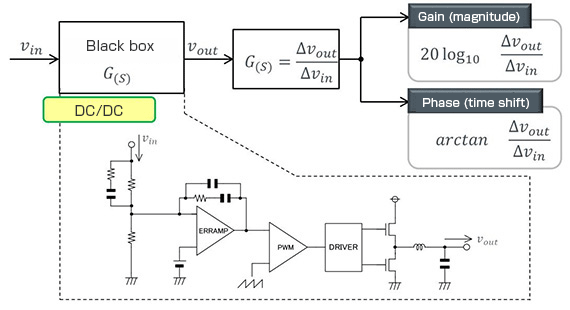Fig. 1

When you hear “gain and phase”, in an analog system, perhaps the first thing that you think of is operational amplifier characteristics. And indeed, when we consider an op-amp as the black box, the gain and phase of the operational amplifier can be represented by a transfer function.

Where DC-DC converters are concerned, measuring the gain and phase characteristics to evaluate the transient response characteristic is well known. A DC-DC converter can likewise be thought of as a black box, and the transfer function representing the gain and phase can be considered. The circuit surrounded by the broken line in Fig. 1 is one example of the interior of the black box in the case of a DC-DC converter.

## Basic approach to derivation of the transfer function

When determining the transfer function on the basis of Fig. 1, a convenient feature of the transfer function can be used: the transfer function can be analyzed into parts, and then described as the product of the parts. A specific example is shown in Fig. 2.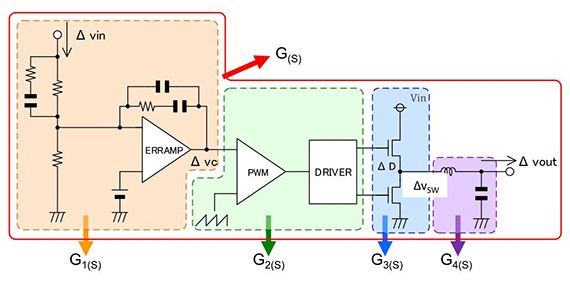Fig. 2

Fig. 2 shows the contents of the black box that is the DC-DC converter of Fig. 1; with G(S) as the entire circuit, enclosed by the red line, the circuit is analyzed into the blocks G1(S) 、G2(S) 、G3(S) 、G4(S) and so can be described as in equation 1-1 below.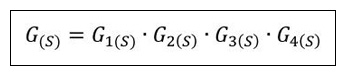Equation 1-1

For example, G1(S) is the transfer function for the error amplifier block, and if for an input of Δvin the output is Δvc, then the transfer function can be represented by Δvc / Δvin, and for each of the blocks the transfer functions can be written as follows.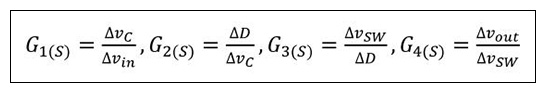Substituting into equation 1-1, we have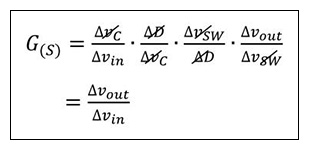Equation 1-2

and it is seen that equation 1-1 is correct.

In other words, by deriving the transfer function for each block, and then taking the product of the transfer functions, the transfer function for the entire circuit can be determined.